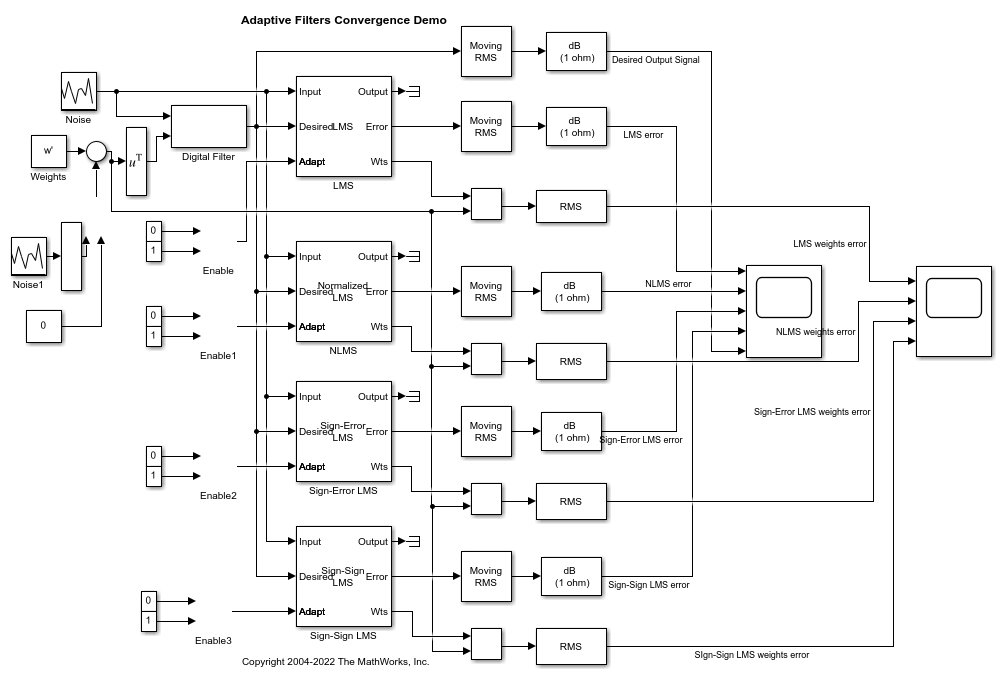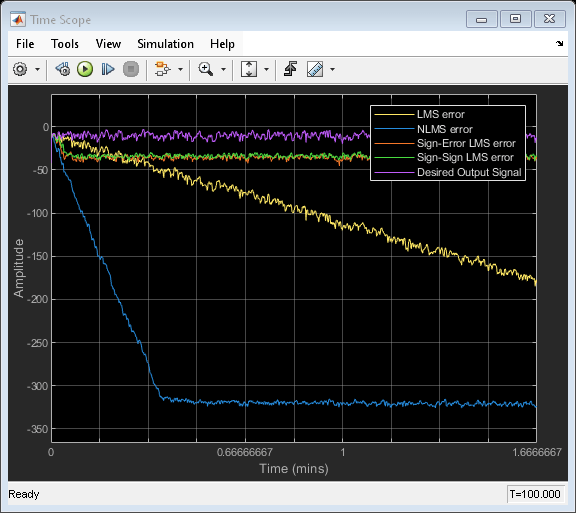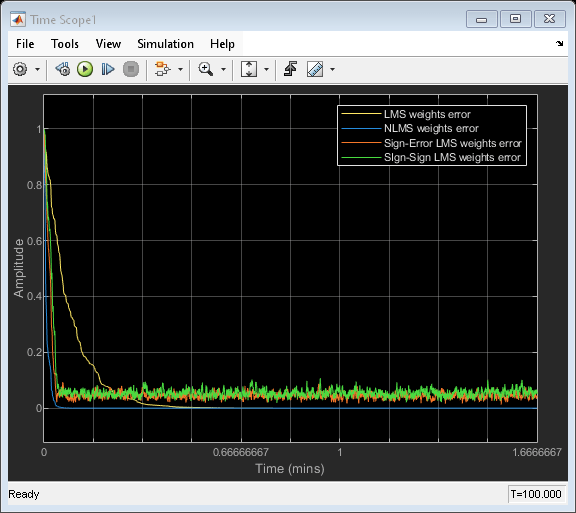This example compares the rate of convergence for adaptive filters using different LMS algorithms.

### Introduction

An adaptive filter adapts its filter coefficients so that its output matches the output of a desired unknown system. A major application of adaptive filters is filter identification, where the reference signal is filtered by an unknown convolution filter, as illustrated here. The same signal is passed through the adaptive filter and the unknown system, and the adaptive filter tries to adjust its coefficients such that the error between the two outputs is minimal. Adaptive filters typically have large error on their outputs initially and the error tends to go down with time, as the filter converges.

Adaptive filters using the Least Mean Squares (LMS) algorithm adjust their coefficients such that the error between the two outputs is minimal in mean square sense. This example considers 4 different variants of the the LMS algorithm and compares the rate of convergence for adaptive filters using these algorithms.

• LMS - Least Mean Square algorithm

• NLMS - Normalized LMS algorithm

• SELMS - Sign-Error LMS algorithm

• SSLMS - Sign-Sign LMS algorithm### Setup

Use a zero mean white Gaussian noise with a variance of 0.01 as the input signal to the filters. Choose an FIR filter of length 13 with all coefficients set to 1 as the desired unknown filter. You can optionally add a Gaussian noise of variance 1e-5 to these weights by toggling the Manual Switch. Set the switch towards the Constant block of value 0 for now, so that the weights do not contain any noise. Set the initial weights of the adaptive filters to 0. Use a step size of 0.2 for the LMS, NLMS and Sign-Error LMS blocks. For the Sign-Sign LMS algorithm, choose a more suitable step size of 0.02. Each of the adaptive filters can be enabled or disabled separately. Use a Moving RMS block to compute the energy of the desired output and the error signals. Visualize these signals in dB scale on a Time Scope. To examine the convergence of the adaptive filter weights, subtract the adaptive filter weights from the desired filter weights and plot the error using another Time Scope.From the first plot, it can be seen that the error signal from the NLMS filter converges to zero much faster than the other variants, with a steady state error of more than 300 dB. The LMS filter is also able to match the output of the unknown filter closely with an SNR of more than 150 dB. From the second plot, the weights of the NLMS and LMS filters are also observed to converge to the desired filter weights with minimal final error between the weights.The Sign-Error LMS and Sign-Sign LMS filters appear to start converging faster than the LMS filter initially, but these filters have a larger steady state error in the filter output (SNR of approximately 20 dB) and filter weights.

## SupportGet trial now